# Covering a square with unit squares

 Importance: Medium ✭✭
 Author(s):
 Subject: Geometry
 Keywords:
 Posted by: Martin Erickson on: July 18th, 2011
Conjecture   For any integer, it is impossible to cover a square of side greater thanwith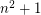unit squares.

Alexander Soifer in [S] raises the question of the smallest numberof unit squares that can cover a square of side. He shows the asymptotic upper bound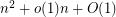, and the small values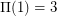,, and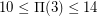. He conjectures the asymptotic lower bound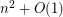.

## Bibliography

[S] Soifer, Alexander, "Covering a square of side n+epsilon with unit squares," J. of Combinatorial Theory, Series A 113 (2006):380-383.

* indicates original appearance(s) of problem.

### A lower bound of the upper bound from polyomino-covering in [S]

(Usinginstead of)
In [S], Soifer derives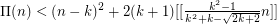.
As he mentioned, one can improve the covering construction. Holding the square of side lengthin the lower left corner, putting a square of side lengthin the upper right corner, covering the remaining uncovered area by 2 polyomino-coverings of rectangles of sidesby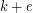, removing useless unit squares in polyominos, we get a lower bound for the rhs of that inequality: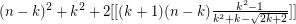Denote bythe minimal value of this expression when varyingfrom 2 to.
Results of computer calculations:iff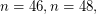or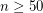.
For growing(checked up to), for the lowest optimal,seems to converge to 1, andseems to converge to 3/4.

### A flaw in the text of the conjecture

The square to cover is not a unit square.

### The author has removed the flaw

... by a small revision.

### A simple upper bound for Pi(n)

For any positive integer n: Pi(n) does not exceed sqr(n)+n+1 .
(Sketchy) proof, using e instead of epsilon:
To cover the square of side length n+e :
Place n by n unit squares as a square of side length n in the lower left corner. Move those unit squares on the upper-right side of the diagonal running from the upper left to the lower right corner by e up and right.
Now we have one set of unit squares in the lower left and one in the upper right corner. The remaining uncovered area is a Zigzag-path of width e consisting of n+1 horizontal lines of length 1+e and n vertical lines of length 1-e. If e is small enough, it is possible to cover that area with a regular array of n+1 non-overlapping unit squares such that each of them covers one horizontal line and parts of the one or two connected vertical lines.

### Correction

Sorry; please replace the last part by this:
The remaining uncovered area is a Zigzag-path of width e consisting of n horizontal lines of length 1+e, n-1 vertical lines of length 1-e, and one vertical line of length 1. If e is small enough, it is possible to cover that area with a regular array of n+1 touching but not (2-dimensional) overlapping unit squares such that each of the first n of them covers one horizontal line and parts of the one or two connected vertical lines and the remaining square covers the remaining part of the (lower) vertical line.

### Resulting upper bounds for n from 1 to 3

The given bound confirms the bounds for n=1 (3) and for n=2 (7) given by Soifer in [S] but improves the bound for n=3 (13 instead of 14).

The two articles listed below may be on the same topic but I can't get access even to the abstracts:  Title: A Sharper Upper Bound for Cover-Up Squared Authors: Dmytro Karabsh and Alexander Soifer Publication: Geombinatorics Quarterly Vol XVI, Issue 1, July 2006 Pages: 219 ff. (to 226 ?)  Title: Note on Covering Square with Equal Squares Authors: Dmytro Karabsh and Alexander Soifer Publication: Geombinatorics Quarterly Vol XVIII, Issue 1, July 2008 Pages: 13 ff. (to 17 ?)

### Correction

The first author's name is Karabash.

### A (currently) valid link to the referenced article

www.uccs.edu/~faculty/asoifer/docs/untitled.pdf

### Correction

In the URL there has to be a tilde (ASCII code 126) between 'edu/' and 'faculty' instead of the visible blank.